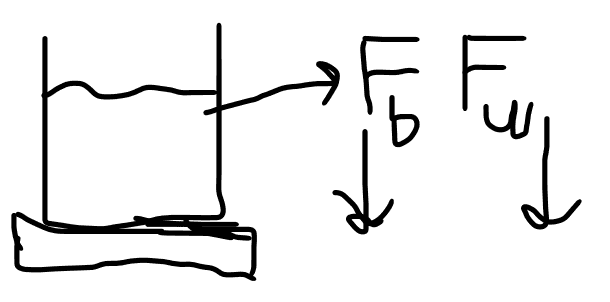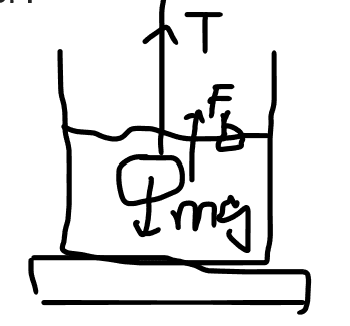# Exploring the Relationship Between Buoyancy and Apparent Mass

• TkoT

#### TkoT

Homework Statement
A cylindrical beaker of mass mb = 1.3 kg contains 1.5 x 10^3 ml of water. The beaker is placed on a scale and then a rock of mass mr = 2.2 kg, suspended by a massless string, is totally immersed in the water. The water level rises by 1.5 cm. The diameter of the beaker is 0.2m
a) What mass does the scale measure before the rock is lowered into the water?
b) What mass does the scale measure after the rock is lowered into the water?
Relevant Equations
F=mg
my solution:
a)F(upward)=Fb +Fw
=(1.3+1.5)X9.8
=27.44N
total Mass = 2.8kg
b)Volume increased = π(0.2/2)^2 x 1.5/100
=4.7x10-4 m^3

T+Fb =mg
T=mg-Fb
T=2.2x9.8 -1000 x 4.7x10-4 x 9.8
T=17.4N
T is the apparent weight of the rock, so the mass of the rock in the water is 1.74kg
So, the total mass measured by the scale = 1.74 +2.8 = 4.54kg

Question:
I am confused about the part b after I checked the answer. For me, I think the tension represents the apparent weight of the rock. So apparent mass of the rock can be obtained by the tension. But, In the answer, buoyant force is considered as the extra weight added to the scale. That confuses me and I don’t understand why.

#### Attachments

Last edited:
Homework Statement:: A cylindrical beaker of mass mb = 1.3 kg contains 1.5 x 10^3 ml of water. The beaker is placed on a scale and then a rock of mass mr = 2.2 kg, suspended by a massless string, is totally immersed in the water. The water level rises by 1.5 cm. The diameter of the beaker is 0.2m
a) What mass does the scale measure before the rock is lowered into the water?
b) What mass does the scale measure after the rock is lowered into the water?
Relevant Equations:: F=mg

That confuses me and I don’t understand why.
Without the rock the scale reads just the weight of the water, ##m_{\text{water}}g##. When the rock is placed under water, the water exerts buoyant force BF up. By Newton's 3rd law, the rock exerts force BF down on the water. The scale reads the sum of the two because it must exert normal force ##N=BF+m_{\text{water}}g## up to keep the water from accelerating.

•TkoT
You seem to be using 9.8m/s2 for g in some places and 10m/s2 in others.